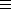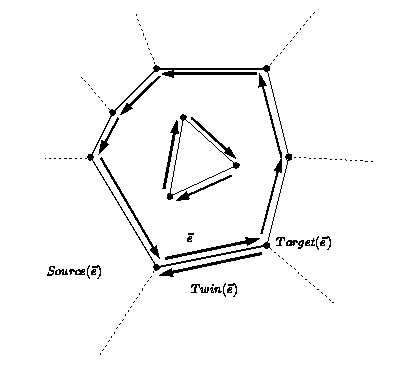# Chapter 18Topological Maps

Iddo Hanniel and Shai Hirsch

### Introduction

A topological map is a graph which consists of vertices, edges, faces and an incidence relation on them. Each edge is represented by two halfedges with opposite orientations. A face of the topological map is defined by the ordered circular sequences (inner and outer) of halfedges along its boundary.

We consider each edge e to be two-sided, representing it by two directed halfedges e and Twin(e) (In other packages the twin halfedge is called Opposite). A halfedge e is an ordered pair (u,v) of its endpoints, and it is directed from u, the source, to v, the target (there is no need to store both in each halfedge since Target(e)Source(Twin(e)).) We consider each halfedge to lie on the boundary of a single face. Each connected component of the boundary of a face is defined by a circular list of halfedges.

For a face f of a topological map, we call each connected component of the boundary of f a CCB. A bounded face has a unique CCB that is defined to be its outer-CCB. An unbounded face does not have an outer boundary. In the topological map we have one unbounded face.

Every maximal set of halfedges that share the same target can be viewed as a circular list of halfedges ordered around their target vertex.

For a topological map, its DCEL representation consists of a connected list of halfedges for every CCB of every face in the subdivision, with additional incidence information that enables us to traverse the subdivision. For each halfedge the DCEL stores a pointer to its twin halfedge and to the next halfedge around its incident face (see Figure). In addition, for each halfedge the DCEL stores a pointer to the incident face and the target vertex. For each face the DCEL stores a pointer to a halfedge representing its outer-CCB and an iterator over pointers to halfedges representing its inner-CCBs (traversing over a CCB is thus done with repetitive calls to the next halfedge pointer). For each vertex the DCEL stores a pointer to an incident halfedge.

The DCEL is a low-level container class that stores the objects. The topological map layer adds high-level functions and protection of combinatorial validity. Iterators, handles and circulators are also introduced in this layer (pointers are no longer visible in this layer).

In the following specifications, we implement the subdivision by a DCEL. Read about the concept TopologicalMapDcel for a specification of the requirements for a DCEL in our implementation.

Figure:  Source and target vertices, and twin halfedges